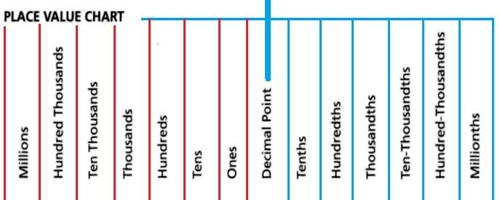Place Values of Decimals

Decimals are numbers with one visible point or dot somewhere in the number. This point is called the decimal point. If a number has a decimal point, then the first digit to the right of the decimal point indicates the number of tenths. A place value tells you the location of each digit in a number. The number 699, for example, has a 9 in the units place, a 9 in the tens place and a 6 in the hundreds place. All numbers follow the same place value naming criteria.

Decimal numbers, such as 0.6495, have four digits after the decimal point. Each digit is a different place value. The first digit after the decimal point is called the tenths place value. There are six tenths in the number 0.6495. The second digit tells you how many hundredths there are in the number. The number 0.6495 has four hundredths. The third digit is the thousandths place.

The fourth digit is the ten-thousandths place which is five in this example.

Therefore, there are six tenths, four hundredths, nine thousandths, and five ten-thousandths in the number 0.6495.Place value and expanded form

6,702 = 6 thousands and 7 hundreds and 0 tens and 2 ones

= 6 × 1000 + 7 × 100 + 0 × 10 + 2 × 1

=  6000 + 700 + 2.

Above, we have written the number 6,702 in expanded form, or as a SUM of its different parts according to place value.

Explanation:

The digit 6 in the number 6,702 actually has the value 6,000 and the digit 7 actually signifies the value 700. This is why our number system is also called a place value system, because the value of a digit (like 6 or 7 in our example) depends on its placement within the number. In other words, the digit 6 in 6702 does not mean six but six thousand, because the six is placed in the thousands’ place. The place of a digit determines its value.

Another explanation: The number 211 has a 1 in the units place, a 1 in the tens place and a 2 in the hundreds place. The number 423 has a 3 in the units place, a 2 in the tens place and a 4 in the hundreds place. Notice how the tens place is always the second digit to the left and that the hundreds place is always the third digit to the left. Believe it or not, you already know most of the place values since you use them to count. Look at this large number broken down into its place values. The number is 123,456.

Information Source: